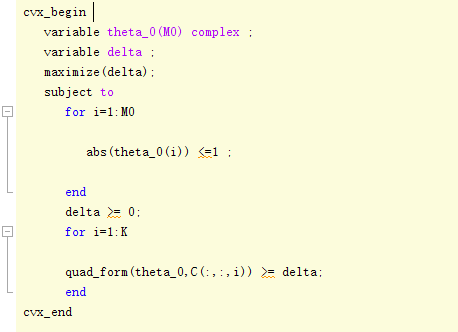# How do I solve the convex objective function maximization

This is a beamforming question. And it’s a convex QCQP problem(C(:,:,i) is positive semidefinite).Here is the errors：The error is clear: the `"quad_.....()"` in your code is convex, but it is in the left side of `>=`, therefore it’s not a convex constraint.

Thx. Could you be more specific? Exactly, this is a max-min problem so that delta was introduced as an auxiliary variable to maximize the lower bound of obejective function. XD

I edited my previous post. Sorry, that statement is not true. Your problem is not convex, so it can’t be expressed with CVX.